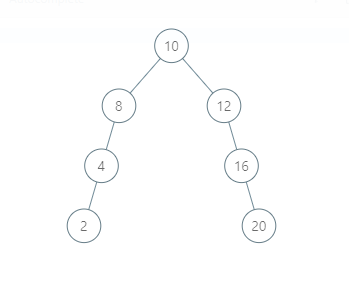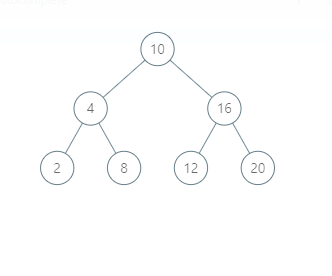New update is available. Click here to update.

# Normal BST To Balanced BST

Last Updated: 3 Dec, 2020
Difficulty: Moderate

## PROBLEM STATEMENT

#### A binary search tree (BST) is a binary tree data structure that has the following properties.

``````• The left subtree of a node contains only nodes with data less than the node’s data.
• The right subtree of a node contains only nodes with data greater than the node’s data.
• Both the left and right subtrees must also be binary search trees.
``````

#### For Example:

``````For the given BST:
````````````The modified BST will be:
``````##### Input Format:
``````The first line contains an integer 'T' which denotes the number of test cases or queries to be run. Then the test cases follow.

The first line of each test case contains the elements of the tree in the level order form separated by a single space.

If any node does not have a left or right child, take -1 in its place. Refer to the example for further clarification.
``````
##### Example:
``````Elements are in the level order form. The input consists of values of nodes separated by a single space in a single line. In case a node is null, we take -1 in its place.

For example, the input for the tree depicted in the below image would be :
````````````1
2 3
4 -1 5 6
-1 7 -1 -1 -1 -1
-1 -1

Explanation :
Level 1 :
The root node of the tree is 1

Level 2 :
Left child of 1 = 2
Right child of 1 = 3

Level 3 :
Left child of 2 = 4
Right child of 2 = null (-1)
Left child of 3 = 5
Right child of 3 = 6

Level 4 :
Left child of 4 = null (-1)
Right child of 4 = 7
Left child of 5 = null (-1)
Right child of 5 = null (-1)
Left child of 6 = null (-1)
Right child of 6 = null (-1)

Level 5 :
Left child of 7 = null (-1)
Right child of 7 = null (-1)

The first not-null node (of the previous level) is treated as the parent of the first two nodes of the current level. The second not-null node (of the previous level) is treated as the parent node for the next two nodes of the current level and so on.

The input ends when all nodes at the last level are null (-1).
``````

#### Note :

``````The above format was just to provide clarity on how the input is formed for a given tree.

The sequence will be put together in a single line separated by a single space. Hence, for the above-depicted tree, the input will be given as:

1 2 3 4 -1 5 6 -1 7 -1 -1 -1 -1 -1 -1
``````
##### Output Format :
``````For each test case, print the inorder traversal of the modified binary tree.

The inorder traversal of a binary tree is the traversal method in which for any node its left subtree is visited first, then the node itself, and then the right subtree.

The output of each test case will be printed in a separate line.
``````

#### Note:

``````You are not required to print anything explicitly. It has already been taken care of. Just implement the function.
``````
##### Constraint :
``````1 <= T <= 100
1 <= N <= 10^5
0 <= data <= 10^8

Where 'data' denotes the value of nodes of BST.

Time Limit: 1 sec
``````